# Algebra II : Basic Statistics

## Example Questions

### Example Question #85 : Basic Statistics

What is the range of the following equation?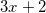where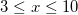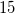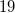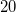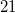Cannot be determinedExplanation:

The range is the smallest value subtracted from the biggest value.

This means that for this specific example you need to plug in "3" into the equation and subtract your answer from what you get when you plug "10" into the equation as these are the two endpoints of your function.

Upon doing this you get: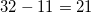### Example Question #51 : Basic Statistics

Find the range of the numbers: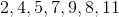.Explanation:

Step 1: Arrange the numbers from smallest to largest: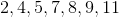.

Step 2: Subtract the largest number and the smallest door to find the range.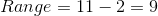The range is.

### Example Question #52 : Basic Statistics

What is the range of the set: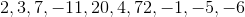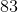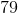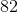Explanation:

Step 1: Rearrange the numbers from smallest to largest:

After rearranging, the set is: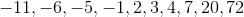.

Step 2: Locate the highest number and the lowest number

Highest=Lowest=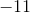Step 3: Subtract Lowest from Highest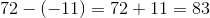The range of this set of numbers is.

### Example Question #53 : Basic Statistics

Solve for the range: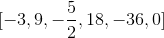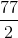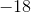Explanation:

The range of a data set is the difference between the highest and lowest numbers.

The highest number is.

The smallest number is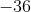.

Subtract the two numbers.  Be sure to enclose the negative number with parentheses.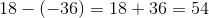The range is:### Example Question #54 : Basic Statistics

Solve for the range: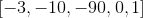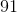Explanation:

The range is the difference between the highest and lowest numbers in the data set.

The highest number is one.

The lowest number is negative ninety.

Subtract both numbers.  Enclose the negative number in parentheses.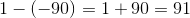The answer is:### Example Question #55 : Basic Statistics

Calculate the range of the data set: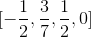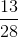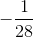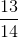Explanation:

The range is the difference between the highest and lowest numbers.

The highest number is:The lowest number is:Subtract both numbers.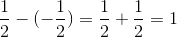The range of the set of data is:### Example Question #56 : Basic Statistics

Solve for the range: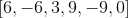Explanation:

The range is the difference between the highest and lowest numbers in the data set.

Determine the highest number.

The highest number is:The lowest number is: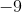Subtract both numbers.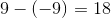The range is:### Example Question #57 : Basic Statistics

Determine the range of the data set: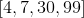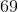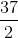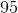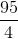Explanation:

The range is the difference between the largest and smallest numbers provided in the set of data.

The highest number is 99, and the smallest number is 4.

Subtract these numbers.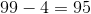The answer is:### Example Question #58 : Basic Statistics

Find the range of the numbers: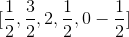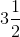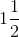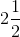Explanation:

The range of a data set the difference between the largest and smallest numbers.

The largest number provided is 2.

The smallest number is negative half.

Subtract these numbers. Make sure to enclose the negative value when subtracting the two numbers.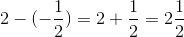The answer is:### Example Question #59 : Basic Statistics

Find the range of the data set: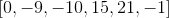Explanation:

The range is the difference between the highest and lowest numbers.

The highest number is 21.

The smallest number is negative 10.

Subtract both numbers.  Make sure to enclose the negative 10 in parentheses.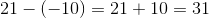The answer is:### All Algebra II Resources